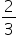Mathematics
Easy

Question

# Find the solution of 3x + 2 = 5x - 1.

## The correct answer is:### To find the solution of the given expression.3x + 2 = 5x - 12x = 3x =Therefore, the value of x is.

### Related Questions to study#### With Turito Foundation.#### Get an Expert Advice From Turito.